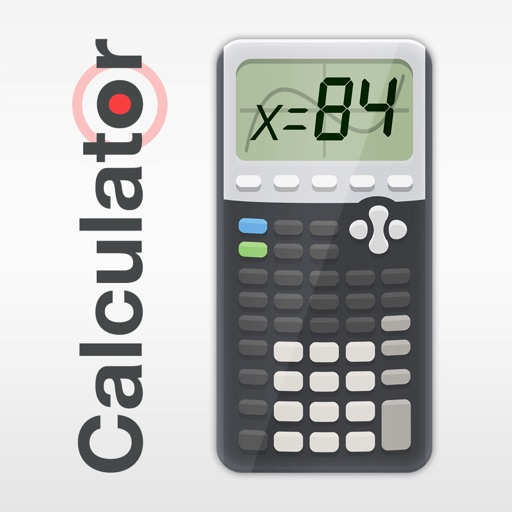## Graphing Calculator X has a formidable reputation of being the FREE alternative to heavy complex hardware calculators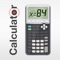# Graphing Calculator X84

by Incpt.Mobis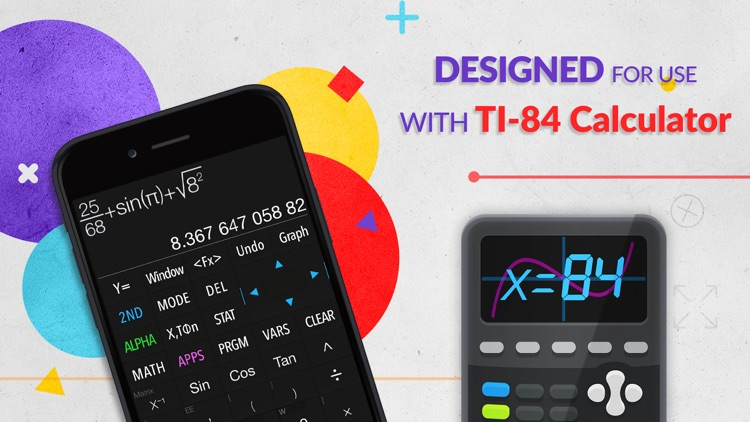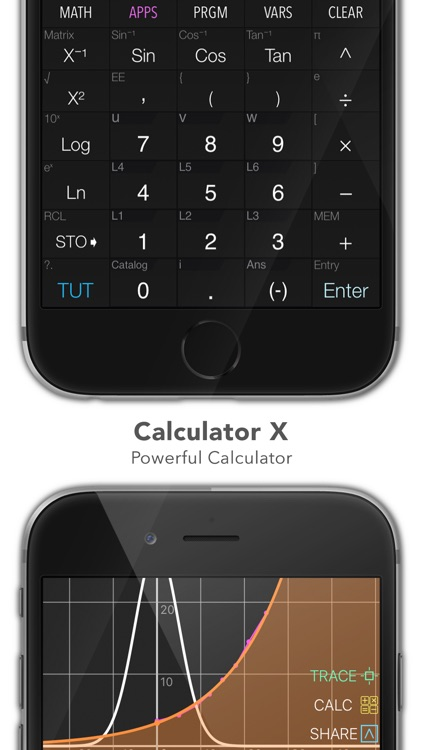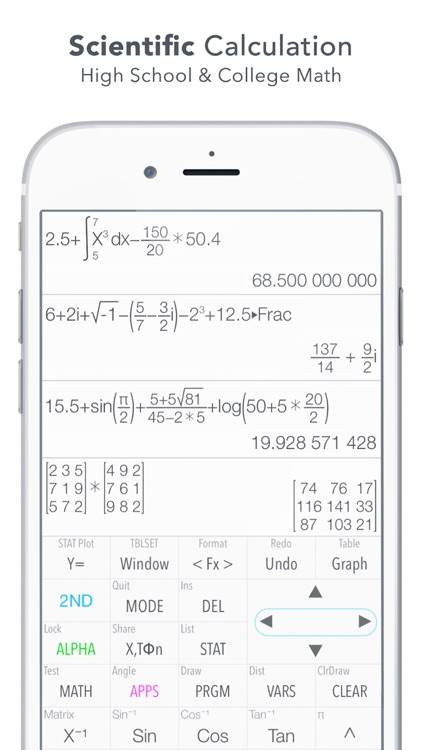Graphing Calculator X has a formidable reputation of being the FREE alternative to heavy complex hardware calculators. It is suitable for all types of users, whether they are looking to carry out basic or complex mathematics; It is equipped with a powerful MATH CORE advocated by MATH DISPLAY support, which increases its functionality by leaps and bounds, making it an ideal choice for engineers, math students etc. It comes with an in depth User Manual and Tutorial to answer any questions you have, regarding the app and to help you further understand the world of advanced mathematics.### App Details

Version
2.7.1
Rating
(20709)
Size
23Mb
Genre
Education Utilities
Last updated
March 23, 2020
Release date
October 17, 2018

### App Screenshots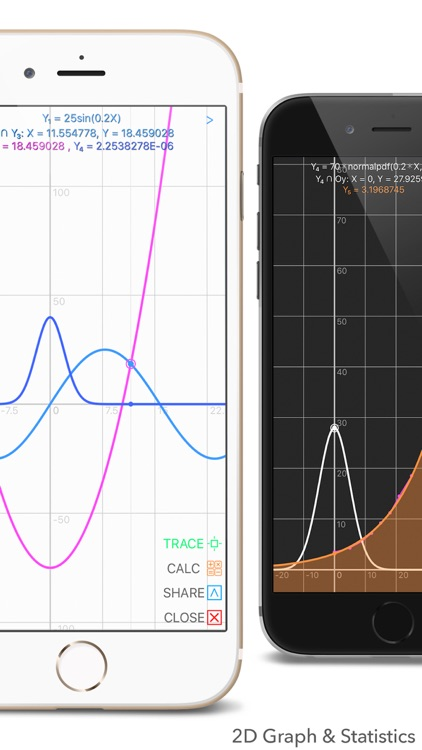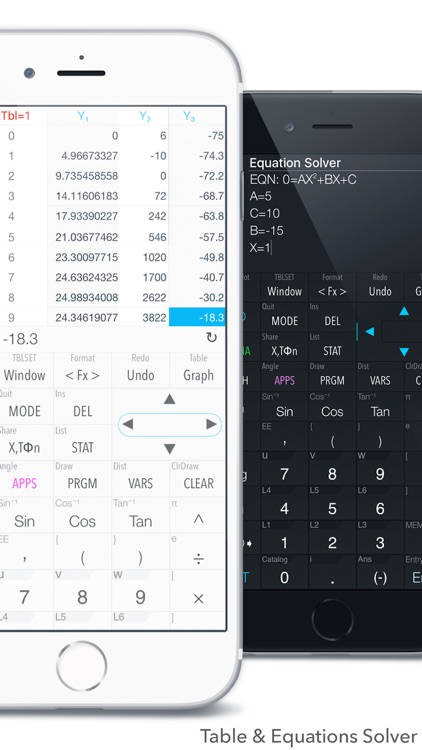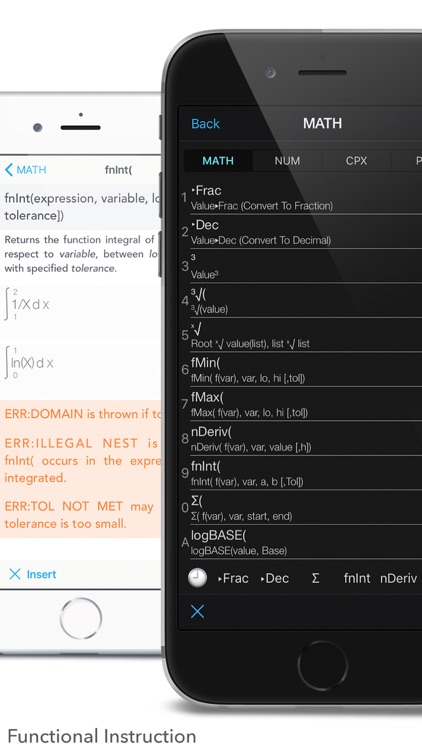### App Store Description

Graphing Calculator X has a formidable reputation of being the FREE alternative to heavy complex hardware calculators. It is suitable for all types of users, whether they are looking to carry out basic or complex mathematics; It is equipped with a powerful MATH CORE advocated by MATH DISPLAY support, which increases its functionality by leaps and bounds, making it an ideal choice for engineers, math students etc. It comes with an in depth User Manual and Tutorial to answer any questions you have, regarding the app and to help you further understand the world of advanced mathematics.

◉ BUILT-IN DOCUMENTS:
▸ Basic General Calculation
▸ X & Y Calculation & Intersection of Functions
▸ Calculate Local Maxima, Minima...
▸ Statistics & Regression
▸ Solve Equations & Polynomial
▸ Calculate Integral Functions

◉ CALCULATOR INCLUDED:
▸ Universal Calculator App
▸ Mathematical Display
▸ Complex Number Calculation
▸ Edit & Export Expression/Result
▸ History Tape View
▸ Basic Calculations (+, -, x, ÷, ^, √,…)
▸ Y-Var & Customize Functions (Y1, Y2)
▸ Fraction and Degree/Angle Calculation
▸ Numeric Integral & Derivative Calculation
▸ Dual Themes(Skin)

◉ 2D GRAPH:
▸ Simultaneous Graph (2 Y-Var)
▸ Smooth Zoom & Transformation
▸ Points TRACING
▸ Box Plot (STAT)

◉ STATISTIC,REGRESSION & TEST:
▸ Create/Edit Lists
▸ 1-Var, 2-Var Statistic
▸ Dialog Wizard Setups

◉ MATRIX:
▸ Create/Edit Matrices
▸ Basic Matrix Functions (+,-,×,÷…)